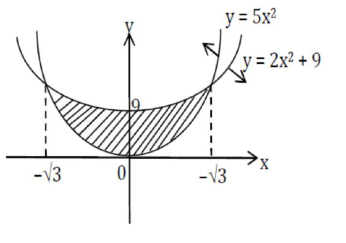# The graphs of sine and cosine functions,

Question:

The graphs of sine and cosine functions, intersect each other at a number of points and between two consecutive points of intersection, the two graphs enclose the same area $A$. Then $A^{4}$ is equal to

Solution:

$Required area$=2 \int_{0}^{\sqrt{3}}\left(2 x^{2}+9-5 x^{2}\right) d x=2 \int_{0}^{\sqrt{3}}\left(9-3 x^{2}\right) d x=2\left|9 x-x^{3}\right|_{0}^{\sqrt{3}}=12 \sqrt{3}\$